# Classifying triangles#### Everything You Need in One Place

Homework problems? Exam preparation? Trying to grasp a concept or just brushing up the basics? Our extensive help & practice library have got you covered.#### Learn and Practice With Ease

Our proven video lessons ease you through problems quickly, and you get tonnes of friendly practice on questions that trip students up on tests and finals.#### Instant and Unlimited Help

Our personalized learning platform enables you to instantly find the exact walkthrough to your specific type of question. Activate unlimited help now!##### Intros
###### Lessons
1. Naming types of triangles based on their properties
1. By angles
• Acute
• Right
• Obtuse
2. By sides
• Scalene
• Isosceles
• Equilateral
##### Examples
###### Lessons
1. Classify the triangle by its sides and angles.

1.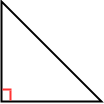2.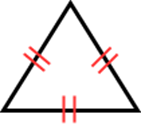3.4.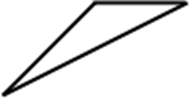2. Draw and classify the triangle by its vertices. Then, determine if it is a right triangle.
1. A(3, 6), B(3, 1), C(9, 1)
2. A(2, 4), B(5, 10), C(5, -2)
3. A(8, 7), B(11, 3), C(4, 2)
3. Find x. Then, classify the triangle by its angles.

1.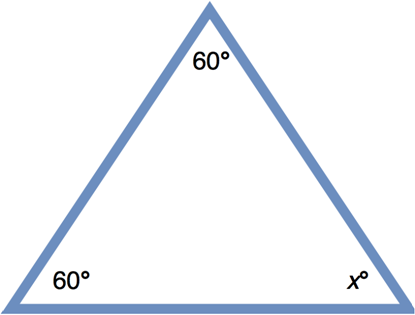2.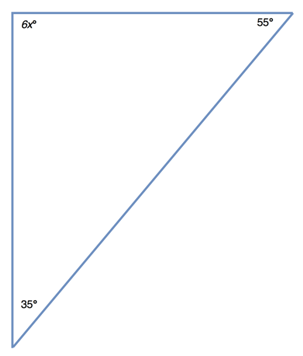3.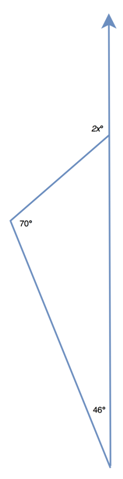4. One angle of a triangular chocolate box is 20°. The measure of the second angle is 3 times smaller than the third one. Find the measures of the two unknown angles.